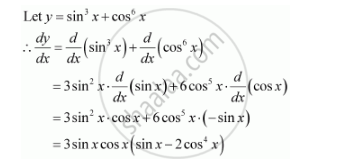Share

# Differentiate W.R.T. X the Function Sin3 X + Cos6 X - CBSE (Commerce) Class 12 - Mathematics

#### Question

Differentiate w.r.t. x the function sin3 x + cos6 x

#### SolutionIs there an error in this question or solution?

#### APPEARS IN

NCERT Solution for Mathematics Textbook for Class 12 (2018 to Current)
Chapter 5: Continuity and Differentiability
Q: 2 | Page no. 191

#### Video TutorialsVIEW ALL 

Solution Differentiate W.R.T. X the Function Sin3 X + Cos6 X Concept: Concept of Differentiability.
S